#### Op-Amp Applications, Physics tutorial

Constant-Gain Multiplier:

One of the most common op-amp circuits is inverting constant-gain multiplier that gives precise gain or amplification. The resulting gain being given by:

A = -Rf/R1

Multiple-stage Gains:

When the numbers of stages are joined in series, overall gain is product of individual stage gains. The first stage is joined to give non-inverting gain. Next two stages give inverting gain. Overall gain is then non-inverting and computed by

A = A1A2A3

Where

A1 = 1 + Rf/R1, A2 = -Rf/R2 and A3 = -Rf/R3

Voltage Summing:

Another popular use of the op-amp is as summing amplifier. The connection with output being sum of three inputs each multiplied by the different gain. Output voltage is

V0 = -((Rf/R1)V1 + (Rf/R2)V2 + (Rf/R3)V3

Voltage Subtraction:

Two signals can be subtracted, one from another, in various ways. Two op-amp stages utilized to give subtraction of input signals. Resulting output is provided by:

V0 = -((Rf/R2)V2 - (Rf/R3)(Rf/R1)V1)

Voltage Buffer:

The voltage buffer circuit gives the means of isolating input signal from load by using stage having unity voltage gain, with no phase or polarity inversion, and acting as ideal circuit with very high input impedance and low output impedance. The op-amp connected to give this buffer amplifier operation. Output voltage is provided by

V0 = V1

Advantage of this connection is that load connected across one output has no (or little) effect on other output. In effect, outputs are buffered or isolated from each other.

Controlled Sources:

Operational amplifiers can be utilized to form various kinds of controlled sources. Input voltage can be utilized to control output voltage or current. These kinds of connections are appropriate for use in different instrumentation circuits.

Voltage-Controlled:

The output voltage is seen to be dependent on input voltage (times a scale factor k). This kind of circuit can be built using op-amp. Two versions of circuit are shown, one using inverting input and other using non-inverting input. The output voltage is:

V0 = -(Rf/R1)V1 = kV1

V0 = (1 + Rf/R1)V1 = kV1

Voltage-Controlled Current Source:

The output current is dependent on input voltage. The practical circuit can be built with output current through load resistor RL controlled by input voltage V1. Current through load resistance RL can be seen to be

I0 = V1/R1 = kV1

Current-Controlled Voltage Source:

The output voltage is dependent on input current. The practical form of circuit is built using the op-amp.

Output voltage is seen to be

V0 = -I1RL = kl1

Current-Controlled Current Source:

In this kind of circuit, output current is provided dependent on input current. Input current I1 can be shown to result in output current I0 so that

I0 = I1 + I2 = I1 + I1R1/R2 = (1 + R1/R2)I1 = kI1

Instrumentation Circuits:

Popular area of op-amp application is in instrumentation circuits like dc or ac voltmeters. Few typical circuits will show how op-amp can be utilized.

DC Millivoltmeter:

The 741 op-amp utilized as basic amplifier in a dc Millivoltmeter. Amplifier gives meter with high input impedance and scale factor dependent only on resistor value and accuracy. The meter reading represents millivolts of signal at circuit input. The analysis of op-amp circuit gives circuit transfer function

|I0/V1| = Rf/R1(1/Rs) = (100kΩ/100kΩ)(1/10kΩ) = 1mA/10mV

Therefore, input of 10 mA will result in the current through meter of 1 mA. If input is 5 mV, current through the meter will be 0.5 mA that is half-scale deflection. Changing Rf to 200kΩ, for instance, would result in circuit scale factor of

|I0/V1| = Rf/R1(1/Rs) = (100kΩ/100kΩ)(1/10kΩ) = 1mA/10mV

Showing that meter now reads 5 mA, full scale. It must be remembered that building such a Millivoltmeter needs purchasing op-amp, a few resistors, diodes, capacitors, and meter movement.

AC Millivoltmeter:

Another example of an instrumentation circuit is ac Millivoltmeter shown below. The circuit transfer function is

|I0/I1| = (Rf/R1)(1/Rs) = (100kΩ/100kΩ)(1/10Ω) = 1mA/10mA

This seems the same as dc Millivoltmeter, except that in this case signal handled is the ac signal. Meter indicator gives a full- scale deflection for ac input signal of 10 mA, while the ac input of 5mA will result in half-scale deflection with meter reading interpreted in millivolts unit.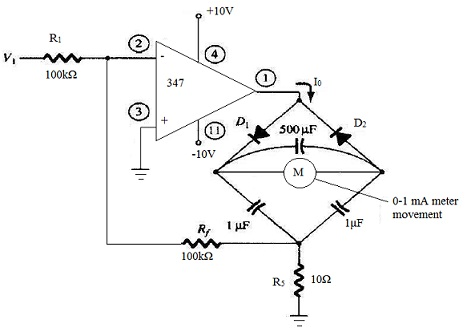Display Divider:

Op-amp circuit can be utilized to drive the lamp display or LED display. When non-inverting input to circuit goes above inverting input, output at terminal 1 goes to positive saturation level (near +5V in this example) and lamp is driven on when transistor Q1 conducts. As shown in circuit, output of op-amp gives 30 mA of current to base of transistor Q1 that then drives 600 mA through properly selected transistor (with β>20) capable of handling that amount of current.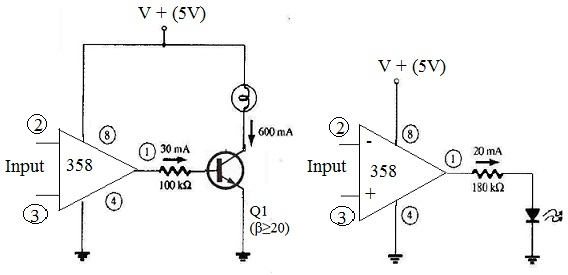Another circuit shows op-amp circuit which can supply 20 mA to drive LED display when non-inverting input goes positive compared to inverting input.

Instrumentation Amplifier:

A circuit giving the output based on difference between two inputs (times a scale factor). Potentiometer is provided to allow adjusting scale factor of circuit. While three op-amps are utilized, a single-quad op-amp IC is all that is essential (other than resistor components.) Output voltage can be shown to be

V0/(V1 - V2) = 1 + 2R/R0

So that the output can be obtained from

V0 = (1 + 2R/R0)(V1 - V2) = k(V1 - V2)

Active Filters:

The popular application uses op-amp to construct active filters. Such amplifiers are developed to amplify only those frequencies which are within certain range. As long as input signal is within specified range, it will be amplified. If it goes outside of this frequency range, amplification will be severely decreased. The tuned amplifier circuits utilizing OP-AMP are usually referred to as active filters. Such circuits don't need use of inductors. Frequency response of circuit is determined by resistor and capacitor values.

Filter circuit can be developed using passive components like resistors and capacitors. But active filter, in addition to passive components makes use of OP-AMP as amplifier. Amplifier in active filter circuit may give voltage amplification and signal isolation or buffering.

There are four main kinds of filters that is, low-pass, filter-high-pass filter, and band-pass filter and band-stop or notch filters.

Low-Pass Filter:

The filter which gives the constant output from dc up to cut-off frequency fOH and then passes no signal above that frequency is known as ideal low-pass filter. Ideal response of low-pass filter is as shown. The response shows that filter has constant output (indicated by the horizontal line AB) from dc or zero frequency up to cut-off frequency (fOH) and beyond fOH, output is zero as indicated by vertical line 'BC' in figure.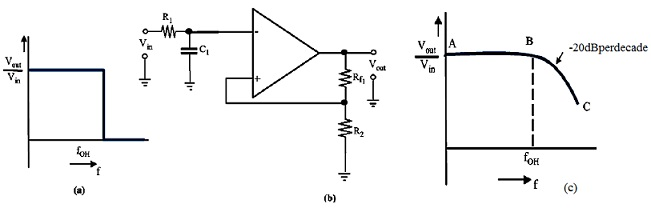The circuit of low- pass active filer using the single resistor and capacitor. Such a circuit is also referred to as first-order (or single- pole) low-pass filter. It is known as first- order as it makes use of single resistor and capacitor. Response of such a first-order low pass filter is shown. Response below cut-off frequency (fOH) shows the constant gain (indicated by horizontal line 'AB'). Though, beyond cut-off frequency, gain doesn't decrease immediately to zero but decreases with slope of 20 dB/decade (means that output voltage decreases by factor of 100 when frequency increases by the factor of 10). Voltage gain for the low-pass filter below cut-off frequency (fOH) is given by relation.

Av = 1 + R3/R1

And cut-off frequency is determined by relation

fOH = 1/2πR4C4

It is possible to connect two sections of filter together. Such a circuit is known as second-order (or two-pole) low pass filter. Second figure shows configuration of second-order low-pass filter.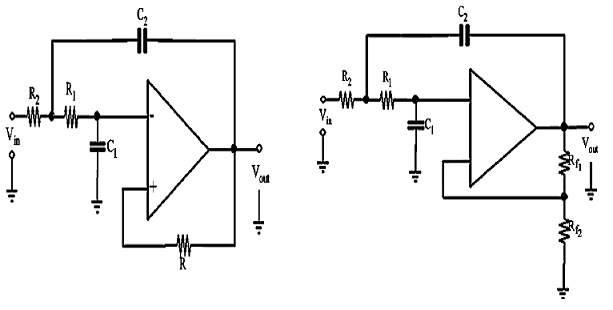Each circuit has two RC circuits, R1 - C1 and R2 - C2. As operating frequency increases beyond f2, each circuit will be dropping closed-loop gain by 20 dB, giving the total roll-off rate of 40dB/decade when operated above f2. Cut-off frequency for each of circuit is given by,

f2 = 1/2π√R1R2C1C2

High-Pass Filter:

As the matter of fact, there is very little difference between high-pass filter and low-pass filter. The circuit of first order (or single-pole) high-pass filter and circuit of the second-order (or two-pole) high-pass filter. Notice that only thing which has changed is position of capacitors and resistors. Value of cut-off frequencies f1 and f2 is obtained by using same equations utilized for low-pass filter.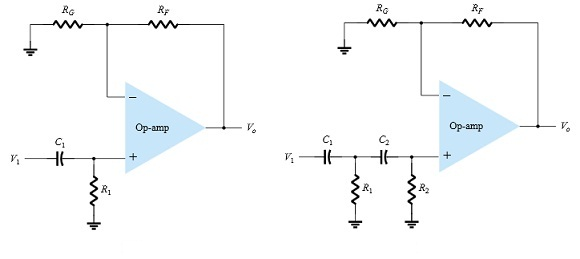The gain versus frequency response of high-pass filter is shown below. Solid line indicates ideal response while dashed line, corresponds to actual response of filter circuit. Ideal curve indicates that filter has a zero output for frequencies below fOL (indicated by line 'AB'). And beyond, it has constant output. Actual response curve may correspond to roll-off gain by 20dB/decade for first order to 40dB/decade for second-order low-pass filters.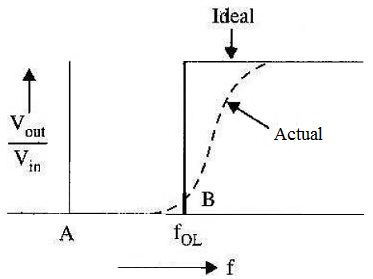Band-Pass Filters:

Band-pass filter is the one which is developed to pass all frequencies within bandwidth. The simple way to create band-pass filter is to cascade low-pass filters and high-pass filter. First stage of band-pass filter will pass all frequencies which are below its cut-off value, f2. All frequencies passed by first stage will head in second stage. This stage will pass all frequencies above value of f1. Result of this circuit action is as shown.

The only frequencies which all will pass through amplifier are those that fall within pass band of both amplifiers. Values of f1 and f2 can be attained by using relations: 1/2πR1C1 and 1/2πR2C2. Then bandwidth, BW = f2 - f1

And center frequency,

f0 = f2 - f1

Quality-factor (or Q-factor) of band-pass filter circuit:

Q = f0/BW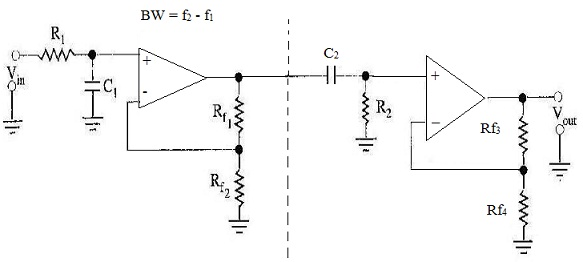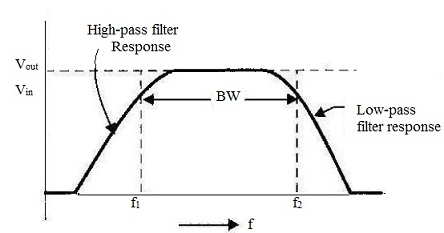Notch Filter:

Notch filter is developed to block all frequencies which fall within bandwidth shows block diagram and, gain versus frequency response curve of the multistage notch filter.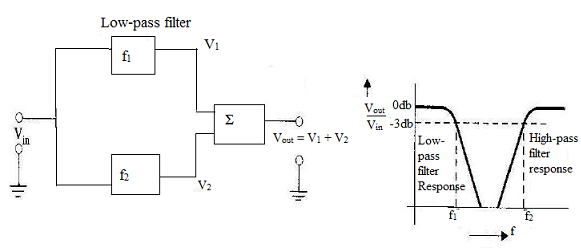Block diagram demonstrates that circuit is composed of high-pass filter, a low-pass filter and assuming amplifier. Summing amplifier generates the output which is equal to sum of filter output voltages. Circuit is developed in such a way so that cut-off frequency, f1 (that is set by low-pass filter) is lower in value than cut-off frequency, f2 (that is set by high-pass filter). Gap between values of f1 and f2 is bandwidth of filter.

When circuit input frequency is lower than f1, input signal will pass through low-pass filter to summing amplifier. As input frequency is below cut-off frequency of high-pass filter, v2 will be zero. Therefore output from summing amplifier will equal output from low-pass filter. When circuit input frequency is higher than, the input signal will pass-through the high-pass filter to summing amplifier. As input frequency is above cut-off frequency of the low-pass filter, v1 will zero. Now summing amplifier output will equivalent to output from high-pass filter.

Frequencies below f1 and those above f2, have been passed by notch filter. But when circuit frequency between f1 and f2, neither of filters will generate output. Thus v1 and v2 will be both zero and output from summing amplifier will also be zero.

Frequency analysis of notch filter is identical to band-pass filter. First, find out cut-off frequencies of low-pass and high-pass filters. Then using these estimated values, find out bandwidth, center frequency and Q values of circuit.

Tutorsglobe: A way to secure high grade in your curriculum (Online Tutoring)

Expand your confidence, grow study skills and improve your grades.

Since 2009, Tutorsglobe has proactively helped millions of students to get better grades in school, college or university and score well in competitive tests with live, one-on-one online tutoring.

Using an advanced developed tutoring system providing little or no wait time, the students are connected on-demand with a tutor at www.tutorsglobe.com. Students work one-on-one, in real-time with a tutor, communicating and studying using a virtual whiteboard technology.  Scientific and mathematical notation, symbols, geometric figures, graphing and freehand drawing can be rendered quickly and easily in the advanced whiteboard.

Free to know our price and packages for online physics tutoring. Chat with us or submit request at info@tutorsglobe.com